#Matlab图像处理-长杆在太阳下的影长度处理方法

0
0
01. 云栖社区>
2. 博客>
3. 正文

## Matlab图像处理-长杆在太阳下的影长度处理方法

pulsar-v 2018-08-29 17:48:03 浏览2332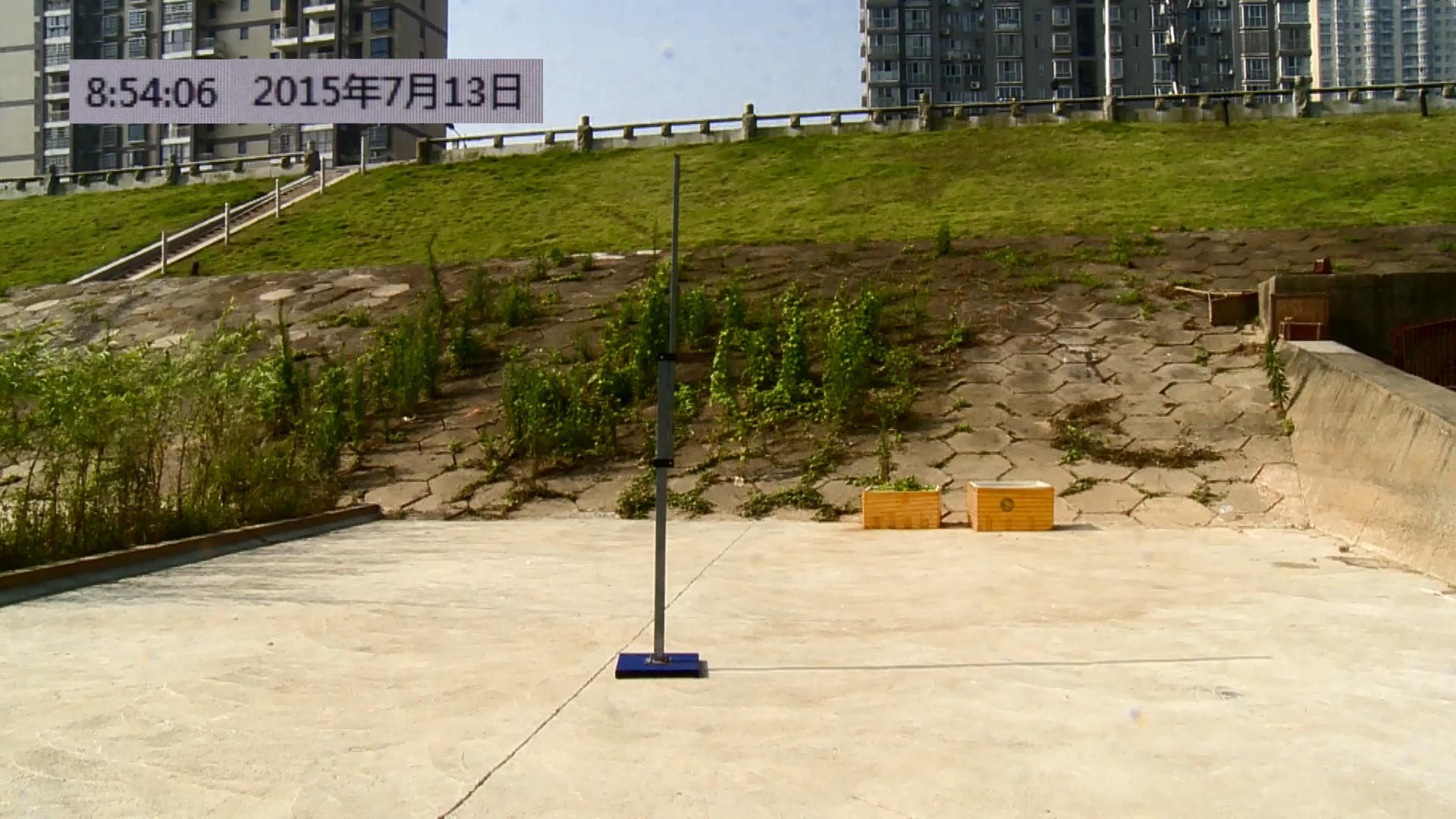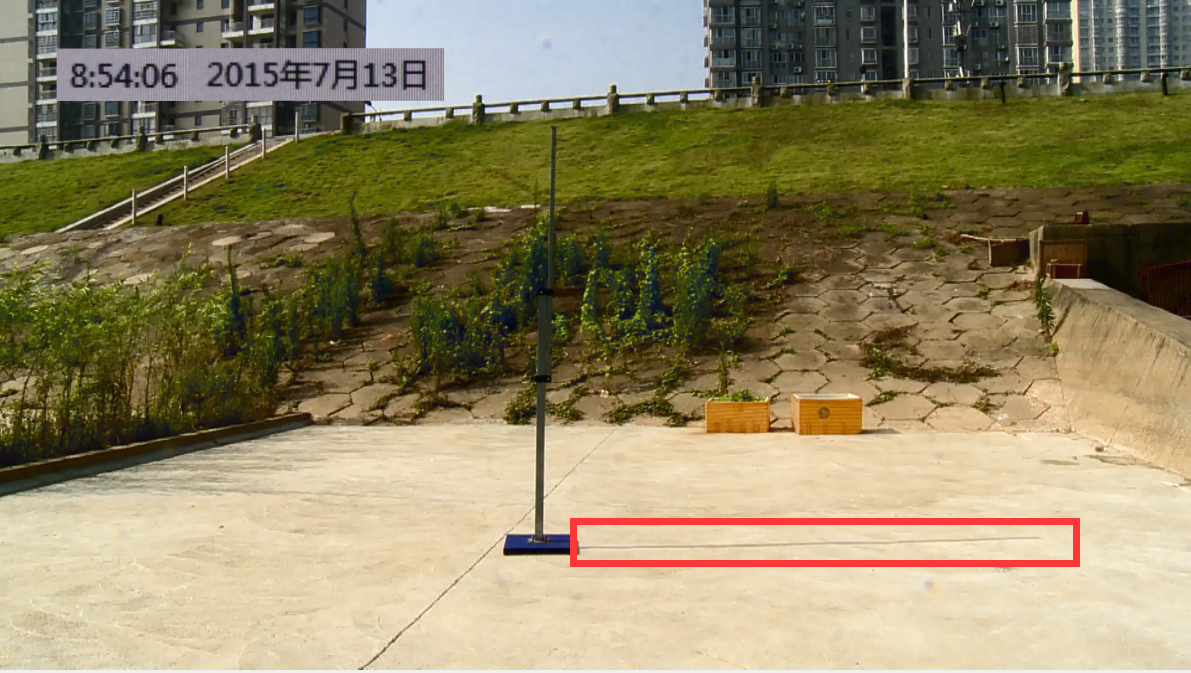## 图像切割拆分

clc
clear
numFrames = obj.NumberOfFrames
for k = 1 :4500: numFrames
imshow(frame);
%imwrite(frame,strcat(num2str(k),'.jpg'),'jpg');
end

## 图像处理

### Canny边缘检测

#### Canny边缘检测算法的处理流程

Canny边缘检测算法可以分为以下5个步骤：

1. 使用高斯滤波器，以平滑图像，滤除噪声。
2. 计算图像中每个像素点的梯度强度和方向。
3. 应用非极大值（Non-Maximum Suppression）抑制，以消除边缘检测带来的杂散响应。
4. 应用双阈值（Double-Threshold）检测来确定真实的和潜在的边缘。
5. 通过抑制孤立的弱边缘最终完成边缘检测。
下面详细介绍每一步的实现思路。

#### 高斯平滑滤波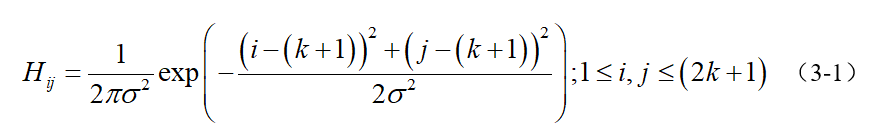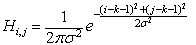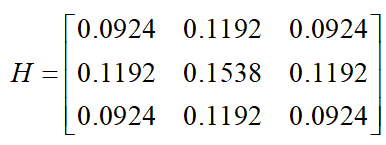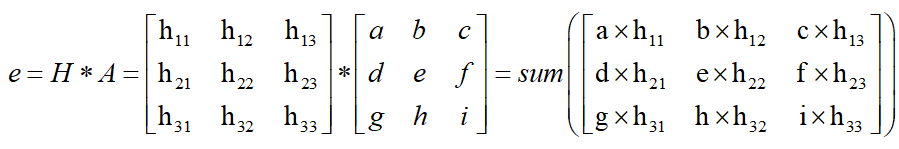#### 计算梯度强度和方向x和y方向的Sobel算子分别为：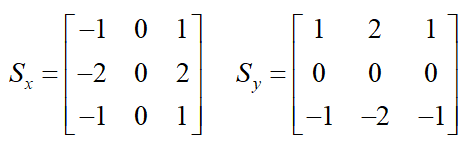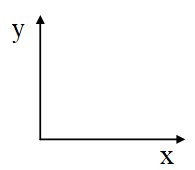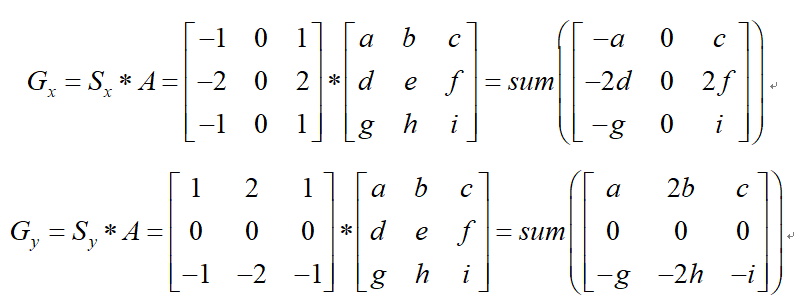#### 非极大值抑制

1. 将当前像素的梯度强度与沿正负梯度方向上的两个像素进行比较。
2. 如果当前像素的梯度强度与另外两个像素相比最大，则该像素点保留为边缘点，否则该像素点将被抑制。
通常为了更加精确的计算，在跨越梯度方向的两个相邻像素之间使用线性插值来得到要比较的像素梯度，现举例如下：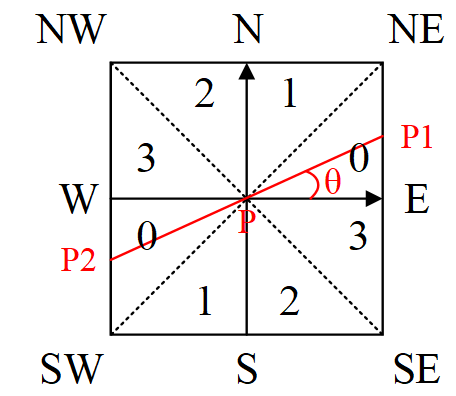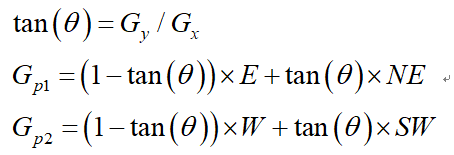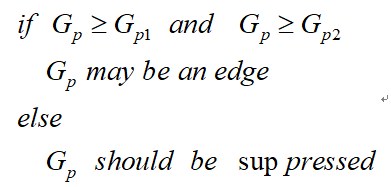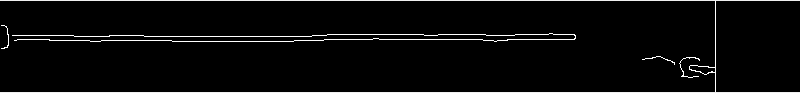clc
clear
count=1
for i=1:4500:58501
%disp(strcat(num2str(i),'.jpg'));
BW=imcrop(BW,[922 850 800 90]);
BW=rgb2gray(BW);
thresh=[0.1,0.17];
sigma=1.4;%定义高斯参数
%Canny边缘检测
f = edge(double(BW),'canny',thresh,sigma);
for i=50:1:91
for j=520:1:900
f(i,j)=0;
end
end
%figure(1),imshow(imcrop(f,[520 40 1000 80]),[]);
[f,x,y]=find_pwite(f);
dis_x(1,count)=x;
dis_y(1,count)=y;
count=count+1;
%imwrite(f,strcat(num2str(count),'.jpeg'),'jpg');
figure(i),imshow(f,[]);
%[x,y,button] = ginput
end

for i=1:length(dis_x);
h(1,i)=power(power(dis_x(1,i),2)+power(dis_y(1,i),2),1/2);
end
t=8.9:0.05:9.55;
plot(t,h,'r');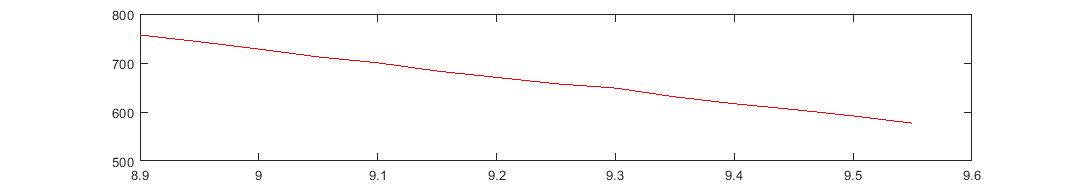pulsar-v
+ 关注